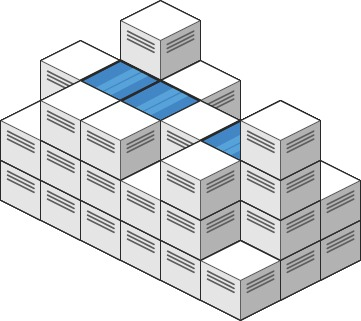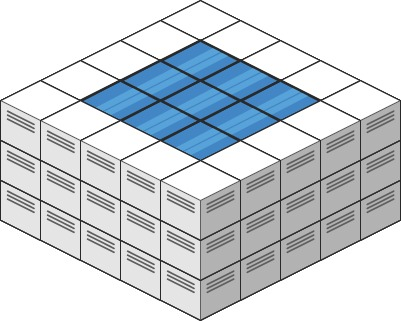# 11. 盛最多水的容器 Container With Most Water

## 例子

输入：[1,8,6,2,5,4,8,3,7]



输入：height = [1,1]



## 提示：

n == height.length

2 <= n <= 10^5

0 <= height[i] <= 10^4

# V1-基本算法

## 思路

## java 实现

java
public int maxArea(int[] height) {
int maxResult = -1;
for(int i = 0; i < height.length; i++) {
for(int j = 0; j < height.length; j++) {
// 跳过自身相等的元素
if(i == j) {
continue;
}
int hi = height[i];
int hj = height[j];

// 高度取最小的
int min = Math.min(hi, hj);

int x = Math.abs(j - i);
int area = x * min;
if(area > maxResult) {
maxResult = area;
}
}
}
return maxResult;
}


## 效果

Runtime: 843 ms, faster than 5.04% of Java online submissions for Container With Most Water.
Memory Usage: 39.4 MB, less than 94.66% of Java online submissions for Container With Most Water.


## 简单优化

/**
* 优化思路
*
* 1. 避免重复计算
* 2. j 永远大于 i，跳过计算
* @param height 高度数组
* @return 结果
* @since v2
*/
public int maxAreaV2(int[] height) {
int maxResult = -1;
for(int i = 0; i < height.length; i++) {
for(int j = i+1; j < height.length; j++) {
int hi = height[i];
int hj = height[j];
int min = Math.min(hi, hj);
int x = j-i;
int area = x * min;
if(area > maxResult) {
maxResult = area;
}
}
}
return maxResult;
}


# V2-双指针法

## 思路

（1）比较二者数字大小

if(height[l] < height[r]) {
// 左边小，那么高度被固定，右边移动已经达到最大。只能左边向右移动
} else {
// 右边下，右边高度被固定，只能最右往左移动。
}


（2）终止条件

l >= R 则终止。

（3）优化点

PS: 实践证明，内部方法确实比 Math 的快。不过一般没必要，这个属于极限优化，一个小技巧。

## java 实现

    public int maxArea(int[] height) {
int l = 0;
int r = height.length-1;
int maxArea = 0;
int width = height.length-1;

while (l < r) {
int area = min(height[l], height[r]) * (width);
maxArea = max(area, maxArea);

// 考虑如何移动指针
if(height[l] >= height[r]) {
// 左边比较大
r--;
} else {
l++;
}
width--;
}
return maxArea;
}

private int min(int a, int b) {
return a < b ? a : b;
}

private int max(int a, int b) {
return a > b ? a : b;
}


## 效果

TC 从 O(N^2) 降低到 O(N)，非常强的算法。

Runtime: 2 ms, faster than 95.28% of Java online submissions for Container With Most Water.
Memory Usage: 40 MB, less than 38.16% of Java online submissions for Container With Most Water.


# 42. 接雨水 Trapping Rain Water

## 题目

### 示例输入：height = [0,1,0,2,1,0,1,3,2,1,2,1]



输入：height = [4,2,0,3,2,5]



n == height.length

1 <= n <= 2 * 10^4

0 <= height[i] <= 10^5

## v1-按列计算

1）第一列和最后一列都是 0

2）中间列的水要怎么求？

### java 实现

    public int trap(int[] height) {
int n = height.length;

// 第一个，最后一列不用看。
int sum = 0;
for(int i = 1; i < n-1; i++) {
int hl = getMaxHeight(height, 0, i-1);
int hr = getMaxHeight(height, i+1, n-1);

int h = Math.min(hl, hr);
if(height[i] < h) {
sum += (h - height[i]);
}
}
return sum;
}

private int getMaxHeight(int[] height,
int startIndex,
int endIndex) {
int res = 0;
for(int i = startIndex; i <= endIndex; i++) {
res = Math.max(res, height[i]);
}

return res;
}


TC: O(N^)

MC: O(1)

321 / 322 执行超时。

## V2-动态规划

### 思路-空间换时间

dp = height;
dp[len-1] = height[len-1];


### java 实现

    public int trap(int[] height) {
int n = height.length;

// 初始化好对应的高度信息。
int[] dpLeft = new int[n];
dpLeft = height;
for(int i = 1; i < n-1; i++) {
dpLeft[i] = Math.max(dpLeft[i-1], height[i]);
}
int[] dpRight = new int[n];
dpRight[n-1] = height[n-1];
for(int i = n-2; i >= 1; i--) {
dpRight[i] = Math.max(dpRight[i+1], height[i]);
}

// 第一个，最后一列不用看。
int sum = 0;
for(int i = 1; i < n-1; i++) {
int hl = dpLeft[i];
int hr = dpRight[i];

int h = Math.min(hl, hr);
if(height[i] < h) {
sum += (h - height[i]);
}
}
return sum;
}


### 效果

TC: O(N)

MC: O(N)

TC: 1ms, 98.34%
MC: 42.8mb, 81.49%


## v3-双指针

### java 实现 1

    public int trap(int[] height) {
int n = height.length;

int[] dpRight = new int[n];
dpRight[n-1] = height[n-1];
for(int i = n-2; i >= 1; i--) {
dpRight[i] = Math.max(dpRight[i+1], height[i]);
}

// 第一个，最后一列不用看。
int sum = 0;
int hl = height;
for(int i = 1; i < n-1; i++) {
hl = Math.max(hl, height[i]);
int hr = dpRight[i];

int h = Math.min(hl, hr);
if(height[i] < h) {
sum += (h - height[i]);
}
}
return sum;
}


### 优化右边高度数组的思路-双指针

left 表示左边当前遍历的柱子（即左边我们需要计算能够装多少水的柱子）

leftMax 表示 left 的左边最高的柱子长度（不包括 left）

right 表示右边当前遍历的柱子

rightMax 表示 right 的右边最高的柱子长度（不包括 right）

？？？？ 为什么 rightMax 和 left 隔这么远我们还可以使用 rightMax 来判断？

rightMax 虽然跟 left 离得远，但有如下两种情况：

1、left 柱子和 rightMax 柱子之间，没有比 rightMax 柱子更高的柱子了，

                        |
|           |
|   |       |
↑   ↑       ↑
l_m  l      r_m


2、left 柱子和 rightMax 柱子之间存在比 rightMax 柱子更高的柱子

left = 1;
right = len - 2;
leftMax = 0;
rightMax = len - 1;


（因为第一个柱子和最后一个柱子肯定不能装水，因为不作为装水柱子，而是作为左边最高柱子和右边最高柱子）

### java 实现

    public int trap(int[] height) {
int n = height.length;

//1. 最大高度
int left = 0;
int right = n-1;
int maxLeft = height[left];
int maxRight = height[right];

int sum = 0;
while (left <= right) {
// 取决于左边
if(maxLeft < maxRight) {
if(height[left] > maxLeft) {
// 无法蓄水
maxLeft = height[left];
} else {
// 可以蓄水
sum += maxLeft - height[left];
}

// 左边指针往右移动
left++;
} else {
// 取决于右边
if(height[right] > maxRight) {
// 无法蓄水
maxRight = height[right];
} else {
// 可以蓄水
sum += maxRight - height[right];
}

// 右边指针往左移动
right--;
}
}

return sum;
}


TC: O(N)

MC: O(1)

# 407. 接雨水 II

## 题目

### 示例输入: heightMap = [[1,4,3,1,3,2],[3,2,1,3,2,4],[2,3,3,2,3,1]]输入: heightMap = [[3,3,3,3,3],[3,2,2,2,3],[3,2,1,2,3],[3,2,2,2,3],[3,3,3,3,3]]



### 提示:

m == heightMap.length

n == heightMap[i].length

1 <= m, n <= 200

0 <= heightMap[i][j] <= 2 * 10^4

## 思路

> [优先级队列与堆排序 PriorityQueue & heap sort](https://houbb.github.io/2019/01/04/prority-queue)

> [图最短路径算法之迪杰斯特拉算法（Dijkstra）](https://houbb.github.io/2020/01/23/data-struct-learn-03-graph-dijkstra)

------------------------------------------------------------------------------------------------------------------------



Given the following 3x6 height map: [ [1,4,3,1,3,2], [3,2,1,3,2,4], [2,3,3,2,3,1] ]





/**

• 把每一个元素称作块。因为那个图片给的好像瓷砖啊。
• 其实做这题一开始都是想的是对于每一个块，去找它四个方向最高的高度中的最小值(二维下则是左右最高的高度取较小的那一个)作为上界，当前块作为下界 但是这4个方向每次遍历复杂度过高，且不能像二维那样去提前预存每个方向的最大值
• 那可以反过来我不以每个块为处理单元，而是以块的四周作为处理单元
• 那如何保证所有四周的可能性都考虑到呢？ 我们从矩阵的最外围往里面遍历，像一个圈不断缩小的过程
• 为了防止重复遍历用visited记录
• 其次要用小顶堆(以高度为判断基准)来存入所有快的四周(即圈是不断缩小的，小顶堆存的就是这个圈)
• 为什么要用小顶堆？ 这样可以保证高度较小的块先出队

** 为什么要让高度较小的块先出队？(关键点)

1. 一开始时候就讲了基础做法是：对于每一个块，去找它四个方向最高的高度中的最小值(二维下则是左右最高的高度取较小的那一个)作为上界，当前块作为下界
2. 而我们现在反过来不是以中心块为处理单元，而是以四周作为处理单元
3. 我们如果能确保当前出队的元素对于该中心块来说是它周围四个高度中的最小值那么就能确定接雨水的大小
4. 为什么队头元素的高度比中心块要高它就一定是中心块周围四个高度中的最小值呢？ 因为我们的前提就是小顶堆：高度小的块先出队，所以对于中心块来说，先出队的必然是中心块四周高度最小的那一个
• 步骤：
1. 构建小顶堆，初始化为矩阵的最外围(边界所有元素)
2. 不断出队，倘若队头元素的四周(队头元素的四周其实就是上面说的中心块，队头元素是中心块的四周高度中最矮的一个) 即代表能够接雨水：队头元素减去该中心块即当前中心块能接雨水的值
3. 但是接完雨水之后中心块还要存进队列中，但这时要存入的中心块是接完雨水后的中心块 */ 

## java 实现

（1）visit 数组，标记是否访问过。

（2）使用优先级队列，初始化的时候，把最外边一圈放进去。保障每次出栈的是最小值

（3）方向处理很巧妙，把四个方向，用一个数组搞定。

（4）处理逻辑：

class Solution {
public int trapRainWater(int[][] heights) {
if (heights == null || heights.length == 0) return 0;
int n = heights.length;
int m = heights.length;
// 用一个vis数组来标记这个位置有没有被访问过
boolean[][] vis = new boolean[n][m];
// 优先队列中存放三元组 [x,y,h] 坐标和高度
PriorityQueue<int[]> pq = new PriorityQueue<>((o1, o2) -> o1 - o2);

// 先把最外一圈放进去
for (int i = 0; i < n; i++) {
for (int j = 0; j < m; j++) {
if (i == 0 || i == n - 1 || j == 0 || j == m - 1) {
pq.offer(new int[]{i, j, heights[i][j]});
vis[i][j] = true;
}
}
}

int res = 0;
// 方向数组，把dx和dy压缩成一维来做
int[] dirs = {-1, 0, 1, 0, -1};
while (!pq.isEmpty()) {
int[] poll = pq.poll();
// 看一下周围四个方向，没访问过的话能不能往里灌水
for (int k = 0; k < 4; k++) {
int nx = poll + dirs[k];
int ny = poll + dirs[k + 1];
// 如果位置合法且没访问过
if (nx >= 0 && nx < n && ny >= 0 && ny < m && !vis[nx][ny]) {
// 如果外围这一圈中最小的比当前这个还高，那就说明能往里面灌水啊
if (poll > heights[nx][ny]) {
res += poll - heights[nx][ny];
}
// 如果灌水高度得是你灌水后的高度了，如果没灌水也要取高的
pq.offer(new int[]{nx, ny, Math.max(heights[nx][ny], poll)});
vis[nx][ny] = true;
}
}
}
return res;
}
}


## 复杂度

TC: O(MNlog(MN))

MC: O(MN) 需要创建额外的空间对元素进行标记

# 开源地址

https://github.com/houbb/leetcode

# 参考资料

https://leetcode.cn/problems/container-with-most-water/

https://leetcode.cn/problems/trapping-rain-water/

https://leetcode.cn/problems/trapping-rain-water/solution/xiang-xi-tong-su-de-si-lu-fen-xi-duo-jie-fa-by-w-8/

https://leetcode.com/problems/trapping-rain-water-ii/description/

https://leetcode.cn/problems/trapping-rain-water-ii/solution/gong-shui-san-xie-jing-dian-dijkstra-yun-13ik/

https://leetcode.cn/problems/trapping-rain-water-ii/solution/you-xian-dui-lie-de-si-lu-jie-jue-jie-yu-shui-ii-b/

https://leetcode.cn/problems/trapping-rain-water-ii/solution/jie-yu-shui-ii-by-leetcode-solution-vlj3/